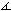# A Sangaku: Two Unrelated Circles

### This applet requires Sun's Java VM 2 which your browser may perceive as a popup. Which it is not. If you want to see the applet work, visit Sun's website at https://www.java.com/en/download/index.jsp, download and install Java VM and enjoy the applet.

 What if applet does not run?

Chords KN and ST are perpendicular to diameter CP of a circle with center O at points Q and R. SQ intersects the circle in V. (K, S are on one side of CP, N and T on the other. Q is between P and R.) Let q be the radius of the circle inscribed into the curvilinear triangle TQV. Prove that

(1)

1/q = 1/PQ + 1/QR.

Solution### Solution

The solution comes from an Art of Problem Solving Forum.

For (1) to hold, the radius q must satisfy

(2)

q = PQ·QR / (PQ + QR) = PQ·QR / PR.

So assume a number q is defined by (2). DenoteQST =QTS = θ. Then QN bisectsTQV andNQV = θ. Place point M on QN at distance q/sinθ from Q. A circle with center M and radius q will touch QV in G, such that MG ⊥ QV. We wish to show that this circle is also tangent to the given one, which, for example, will follow from

(3)

OM = r - q,

where r is the radius of the given circle.

### This applet requires Sun's Java VM 2 which your browser may perceive as a popup. Which it is not. If you want to see the applet work, visit Sun's website at https://www.java.com/en/download/index.jsp, download and install Java VM and enjoy the applet.

 What if applet does not run?

Start by applying the Pythagorean theorem in ΔOQM:

 OM2 = MQ2 + OQ2 = q2/(sinθ)2 + (PQ - r)2 = q2 + q2/(tanθ)2 + (PQ - r)2 = q2 + PQ2·QR2/(PR·tanθ)2 + (PQ - r)2 = q2 + PQ2·SR2/PR2 + PQ2 - 2r·PQ + r2 = q2 + PQ2·(SR2 + PR2)/PR2 - 2r·PQ + r2.

By the Intersecting Chords Theorem,

 SR2 = PR·(2r - PR) = 2r·PR - PR2.

Using this we continue:

 OM2 = MQ2 + OQ2 = q2 + PQ2·(SR2 + PR2)/PR2 - 2r·PQ + r2 = q2 + PQ2·2r·/PR - 2r·PQ + r2 = q2 - 2r·PQ·(PR - PQ)/PR + r2 = q2 - 2r·PQ·QR/PR + r2 = q2 - 2r·q + r2 = (r - q)2,

from which OM = r - q, as expected.

It is noteworthy to observe that the radius in (1) does not depend on the radius r of the given circle, and thus not on point S on the perpendicular PC at R.

Michel Cabart has observed that this problem can be solved by a similar calculation but without first assuming the formula to be true. He proceeds as follows:

We can suppose the circle is of radius 1. Say QP = x, QR = y. Then successively

OQ = 1 - x,
OR = x + y - 1,
QT2 = y2 + [1 - (x + y - 1)2] = 2(x + y) - 2xy - x2,
(sinθ)2 = y2/[ 2(x + y) - 2xy - x2].

By hypothesis OM2 = (1 - q)2 = (1 - x)2 + q2/(sinθ)2.

Move (1 - x)2 to left and divide all by x2q2.

 [(1 - q)2 - (1 - x)2]/x2q2 = [x - q][2 - (q + x)]/ x2q2 (first member) = [2(x + y) - 2xy - x2]/x2y2 (2nd member)

Replace X = 1/x, Y = 1/y, Q = 1/q. First member becomes (Q - X)[2QX - (X + Q)], which by posing Q = X + Y + t becomes

(t + Y) [t(2X - 1) + 2X(X + Y) - 2X - Y] = f(t).

Second member becomes Y[2X(X + Y) - 2X - Y] = f(0).

The trinom f(t) has all its coefficients positive, because

2X - 1 = (2 - x)/x > 0, and
2X(X + Y) - 2X - Y = QT2/x2y2

thus its roots are negative and f(t) is growing when t > 0. So f(t) = f(0) iff t = 0.

A third approach is by Nathan Bowler who starts with the smaller circle:

Take coordinates such that it is the unit circle (q = 1), with Q on the x axis. Let G = (g, h), W = (u, v). Then:

• QV has equation xg + yh = 1.
• Q is at (1/g, 0).
• O is at (1/g, v/ug) = W/ug.
• V satisfies xg + yh = 1 and (x - 1/g)2 + (y - v/gu)2 = (1 - 1/ug)2.

Further y2 - 2ygv/u + 2g/u - g2 - 1 = 0 so that

 QR = -y = -gv/u - sqrt(g2/u2 - 2g/u + 1) = -gv/u + 1 - g/u = 1 - g(1+v)/u.

and

PQ = 1 - 1/ug + v/ug = 1 - (1-v)/ug.

So (PQ - 1)(QR - 1) = (1-v)(1+v)/u2 = 1. Equivalently, 1/PQ + 1/QR = 1 = 1/q.### Mixtilinear Incircles

• Mixtilinear Circles and Concurrence
• Radius and Construction of a Mixtilinear Circle
• Sangaku with Three Mixtilinear Circles
• Construction and Properties of Mixtilinear Incircles
• Construction and Properties of Mixtilinear Incircles 2
• Conic in Mixtilinear Incircles
• A Sangaku: Two Unrelated Circles
•## Sangaku